Courses

# Test: Cross Multiplication Method

## 10 Questions MCQ Test Mathematics (Maths) Class 10 | Test: Cross Multiplication Method

Description
This mock test of Test: Cross Multiplication Method for Class 10 helps you for every Class 10 entrance exam. This contains 10 Multiple Choice Questions for Class 10 Test: Cross Multiplication Method (mcq) to study with solutions a complete question bank. The solved questions answers in this Test: Cross Multiplication Method quiz give you a good mix of easy questions and tough questions. Class 10 students definitely take this Test: Cross Multiplication Method exercise for a better result in the exam. You can find other Test: Cross Multiplication Method extra questions, long questions & short questions for Class 10 on EduRev as well by searching above.
QUESTION: 1

Solution:
QUESTION: 2

### How many solutions of the equation 15x – 14y + 11 = 0 are possible?

Solution:

Since it is a linear equation, and the graph doesn’t end so it gives us infinite solutions, putting different values of x will give different values of y. For example , x = 0  ,y = 11/14  or y = 0, x = -11/15

QUESTION: 3

### Find the value of ‘a” for which the system of equations ax + 2y – 4 = 0 and x – y – 3 = 0 will represent intersecting lines?​

Solution:

The given equations are : ax + 2y – 4 = 0, x – y – 3 = 0is a case of intersecting lines which meet at one.
Here a1=a,a2=1,b1=2,b2=-1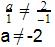QUESTION: 4

For what value of ‘k” is the pair of equations x- ky + 4 = 0 and 2x – 4y – 8 = 0 inconsistent?

Solution:

We have inconsistent equations when the equations are parallel. So we have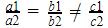x- ky + 4 = 0 and 2x – 4y – 8 = 0
Here,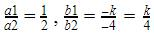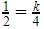k = 2

QUESTION: 5

Which of the following pair of linear equations is inconsistent?​

Solution:
QUESTION: 6

Pair of linear equations x+2y+5 = 0 and 3x+6y -1 = 0 have​

Solution:
QUESTION: 7

Solution for ax + by = a – b and bx – ay = a + b is​

Solution:
QUESTION: 8

For what value of ‘K’ will the system of equations 3x + y = 1, (2K – 1)x + (K – 1)y = 2K + 1 have no solution

Solution:
QUESTION: 9

How many solutions are possible for the following pair of equations? x – y = 1 and 3x – 4y = 4​

Solution:
QUESTION: 10

Find a and b for which (a - 1) x + 3y = 2, 6x + (1 - 2b) y = 6 infinite solution.​

Solution:

Two equations have infinitely many solutions when the lines are coincident lines which means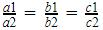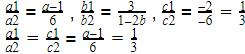a-1=2
a=3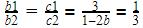1-2b = 9
b = -4